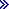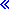# %DIFF (Difference Between Two Date, Time, or Timestamp Values)``%DIFF(op1 : op2 : unit {: frac })``The unit can be *MSECONDS, *SECONDS, *MINUTES, *HOURS, *DAYS, *MONTHS, or *YEARS. You can also use the following abbreviated forms of the unit: *MS, *S, *MN, *H, *D, *M, or *Y.%DIFF produces the difference (duration) between two date or time values. The first and second parameters must have the same, or compatible types. The following combinations are possible:
• Date and date
• Time and time
• Timestamp and timestamp
• Date and timestamp (only the date portion of the timestamp is considered)
• Time and timestamp (only the time portion of the timestamp is considered).
The third parameter specifies the unit. The following units are valid:
• For two dates or a date and a timestamp: *DAYS, *MONTHS, and *YEARS
• For two times or a time and a timestamp: *SECONDS, *MINUTES, and *HOURS
• For two timestamps: *MSECONDS, *SECONDS, *MINUTES, *HOURS, *DAYS, *MONTHS, and *YEARSIf the third operand is *SECONDS or *S, and both of the operands are timestamps, you can specify a fourth parameter indicating the number of fractional seconds to return. You can specify a value between 0 and 12. This represents the number of decimal positions in the returned number of seconds.The difference is calculated by subtracting the second operand from the first.

The result is rounded down, with any remainder discarded. For example, 61 minutes is equal to 1 hour, and 59 minutes is equal to 0 hours.

The value returned by the function is compatible with both type numeric and type duration. You can add the result to a number (type numeric) or a date, time, or timestamp (type duration).If you ask for the difference in microseconds between two timestamps that are more than 32 years 9 months apart, you will exceed the 15-digit limit for duration values. This will result in an error or truncation. However, you can obtain the number of microseconds between any two dates by asking for the difference in seconds with 6 fractional seconds, and then multiply the resulting value by 1000000. For example, if the difference in seconds is 1041379205.123456, then the difference in microseconds is 1041379205123456.Figure 1. Using the result of %DIFF as a numeric value
``````D due_date        S               D   INZ(D'2005-06-01')
D today           S               D   INZ(D'2004-09-23')
D num_days        S             15P 0

D start_time      S               Z

D time_taken      S             15P 0

/FREE

// Determine the number of days between two dates.

// If due_date has the value 2005-06-01 and
// today has the value 2004-09-23, then
// num_days will have the value 251.

num_days = %DIFF (due_date: today: *DAYS);

// If the arguments are coded in the reverse order,
// num_days will have the value -251.

num_days = %DIFF (today: due_date: *DAYS);

// Determine the number of seconds required to do a task:
//  1. Get the starting timestamp
//  3. Calculate the difference between the current
//     timestamp and the starting timestamp

start_time = %timestamp();
process();
time_taken = %DIFF (%timestamp() : start_time : *SECONDS);

/END-FREE``````
Figure 2. Using the result of %DIFF as a duration
``````D estimated_end...
D                 S               D
D prev_start      S               D   INZ(D'2003-06-21')
D prev_end        S               D   INZ(D'2003-06-24')

/FREE

// Add the number of days between two dates
// to a third date

// prev_start is the date a previous task began
// prev_end is the date a previous task ended.

// The following calculation will estimate the
// date a similar task will end, if it begins
// today.

// If the current date, returned by %date(), is
// 2003-08-15, then estimated_end will be
// 2003-08-18.

estimated_end = %date() + %DIFF(prev_end : prev_start : *days);

/END-FREE``````